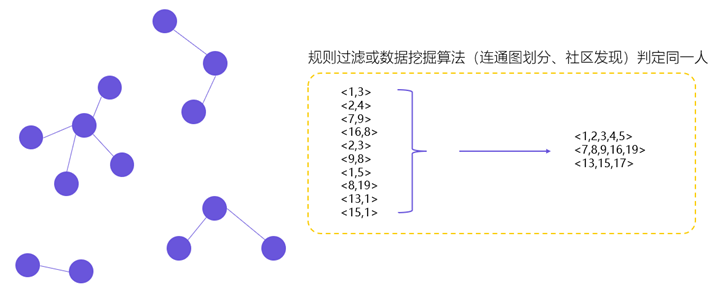# SciPy稀疏矩阵模块scipy.sparse

## scipy.sparse的不同存储格式

Python中scipy模块中，有一个模块叫sparse模块，就是专门为了解决稀疏矩阵而生。导入sparse模块，然后help一把，先来看个大概：

>>> from scipy import sparse
>>> help(sparse)

There are seven available sparse matrix types:

1. csc_matrix: Compressed Sparse Column format
2. csr_matrix: Compressed Sparse Row format
3. bsr_matrix: Block Sparse Row format
4. lil_matrix: List of Lists format
5. dok_matrix: Dictionary of Keys format
6. coo_matrix: COOrdinate format (aka IJV, triplet format)
7. dia_matrix: DIAgonal format


## coo_matrix

coo_matrix是最简单的存储方式。采用三个数组row、col和data保存非零元素的信息。这三个数组的长度相同，row保存元素的行，col保存元素的列，data保存元素的值。一般来说，coo_matrix主要用来创建矩阵，因为coo_matrix无法对矩阵的元素进行增删改等操作，一旦矩阵创建成功以后，会转化为其他形式的矩阵。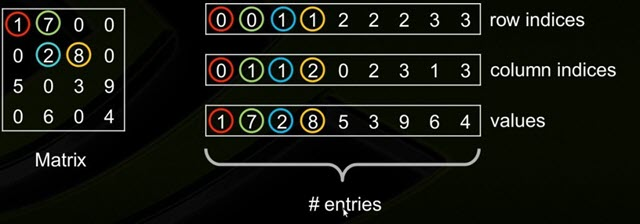from scipy import sparse

row = [1, 2, 0]
col = [0, 0, 3]
data = [4, 0, 5]
c = sparse.coo_matrix((data, (row, col)), shape=(3, 4))
print(c.toarray())
# output
# [[0 0 0 5]
#  [4 0 0 0]
#  [0 0 0 0]]


## csr_matrix 与 csc_matrix

csr_matrix，全名为Compressed Sparse Row，是按行对矩阵进行压缩的。CSR需要三类数据：数值，列号，以及行偏移量。CSR是一种编码的方式，其中，数值与列号的含义，与coo里是一致的。行偏移表示某一行的第一个元素在values里面的起始偏移位置。CSR由三个数组决定:values,columns,row_ptr

• 数组values：保存非0实数，按行优先保存(即从0行到最后一行，每一行从左到右的顺序)
• Columns: 和values长度相同，给出每个非0值所在的列标
• Row_ptr: 长度为稀疏矩阵的行数，保存稀疏矩阵的每行第一个非零元素在values的索引。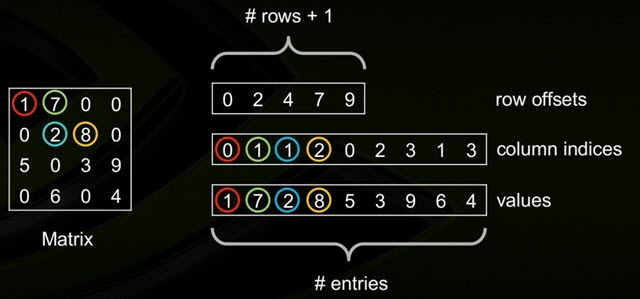from scipy.sparse import csr_matrix

indptr = np.array([0, 2, 3, 6])
indices = np.array([0, 2, 2, 0, 1, 2])
data = np.array([1, 2, 3, 4, 5, 6])
csr_matrix((data, indices, indptr), shape=(3, 3)).toarray()

# output
# array([[1, 0, 2],
#        [0, 0, 3],
#        [4, 5, 6]])


## bsr_matrix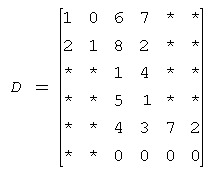block_size为2时，分块表示的压缩矩阵E：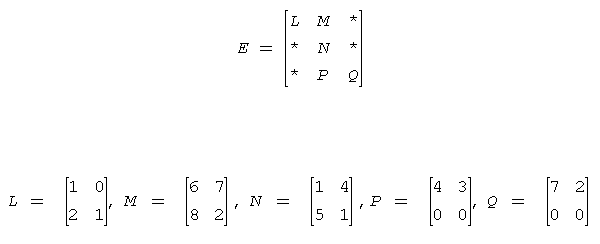BSR的zero-based索引表示：

• values = (1 0 2 1 6 7 8 2 1 4 5 1 4 3 0 0 7 2 0 0)
• columns = (0 1 1 1 2)
• pointerB = (0 2 3)
• pointerE = (2 3 5)

• 数组values：是一个实（复）数，包含原始矩阵A中的非0元，以行优先的形式保存；
• 数组columns：第i个整型元素代表块压缩矩阵E中第i列；
• 数组pointerB ：第j个整型元素给出columns第j个非0块的起始位置；
• 数组pointerE：第j个整型元素给出columns数组中第j个非0块的终止位置

## dia_matrix

DIA通过两个数组确定： values、distance。其中：

• values：对角线元素的值；
• distance：第i个distance是当前第i个对角线和主对角线的距离。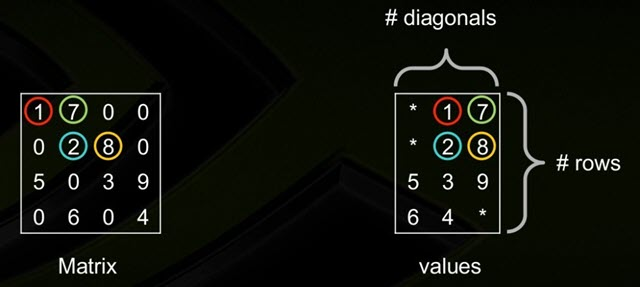## dok_matrix与lil_matrix

dok_matrix和lil_matrix适用的场景是逐渐添加矩阵的元素。doc_matrix的策略是采用字典来记录矩阵中不为0的元素。自然字典的key存的是记录元素的位置信息的元祖，value是记录元素的具体值。

import numpy as np
from scipy.sparse import dok_matrix

S = dok_matrix((4, 4), dtype=np.float32)
for i in range(4):
for j in range(4):
S[i, j] = i + j

print(S.toarray())
# output
# [[0. 1. 2. 3.]
#  [1. 2. 3. 4.]
#  [2. 3. 4. 5.]
#  [3. 4. 5. 6.]]


lil_matrix则是使用两个列表存储非0元素。data保存每行中的非零元素,rows保存非零元素所在的列。这种格式也很适合逐个添加元素，并且能快速获取行相关的数据。

from scipy.sparse import lil_matrix

l = lil_matrix((6, 5))
l[2, 3] = 1
l[3, 4] = 2
l[3, 2] = 3
print(l.toarray())
print(l.data)
print(l.rows)
# output
# [[0. 0. 0. 0. 0.]
#  [0. 0. 0. 0. 0.]
#  [0. 0. 0. 1. 0.]
#  [0. 0. 3. 0. 2.]
#  [0. 0. 0. 0. 0.]
#  [0. 0. 0. 0. 0.]]
# [list([]) list([]) list([1.0]) list([3.0, 2.0]) list([]) list([])]
# [list([]) list([]) list() list([2, 4]) list([]) list([])]


## 不同压缩存储格式的优缺点

• BSR-分块压缩矩阵。BSR更适合于有密集子矩阵的稀疏矩阵，分块矩阵通常出现在向量值有限的离散元中，在这种情景下，比CSR和CSC算术操作更有效。
• CSC-列压缩格式。高效的CSC +CSC,CSC*CSC算术运算；高效的列切片操作。但是矩阵内积操作没有CSR,BSR快；行切片操作慢（相比CSR）；稀疏结构的变化代价高（相比LIL 或者 DOK）。
• CSR-行压缩格式。高效的CSR + CSR, CSR *CSR算术运算；高效的行切片操作；高效的矩阵内积内积操作。但是列切片操作慢（相比CSC）；稀疏结构的变化代价高（相比LIL 或者 DOK）。CSR格式在存储稀疏矩阵时非零元素平均使用的字节数(Bytes per Nonzero Entry)最为稳定（float类型约为5，double类型约为12.5）。CSR格式常用于读入数据后进行稀疏矩阵计算。
• DIA-对角压缩。对角存储格式(DIA)和ELL格式在进行稀疏矩阵-矢量乘积(sparse matrix-vector products)时效率最高，所以它们是应用迭代法(如共轭梯度法)解稀疏线性系统最快的格式；DIA格式存储数据的非零元素平均使用的字节数与矩阵类型有较大关系，适合于StructuredMesh结构的稀疏矩阵（float类型约为05，double类型约为8.10）。对于Unstructured Mesh以及Random Matrix，DIA格式使用的字节数是CSR格式的十几倍。

• COO和CSR格式比起DIA和ELL来，更加灵活，易于操作；
• ELL的优点是快速，而COO优点是灵活，二者结合后的HYB格式是一种不错的稀疏矩阵表示格式；
• CSR格式在存储稀疏矩阵时非零元素平均使用的字节数(Bytes per Nonzero Entry)最为稳定（float类型约为5，double类型约为12.5），而DIA格式存储数据的非零元素平均使用的字节数与矩阵类型有较大关系，适合于StructuredMesh结构的稀疏矩阵（float类型约为4.05，double类型约为8.10，对于Unstructured Mesh以及Random Matrix,DIA格式使用的字节数是CSR格式的十几倍；
• 一些线性代数计算库：COO格式常用于从文件中进行稀疏矩阵的读写，如matrix market即采用COO格式，而CSR格式常用于读入数据后进行稀疏矩阵计算。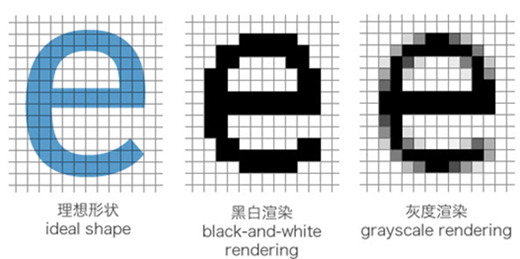##### 网站与APP开发中的字体设置##### 如何理解Python装饰器